# ANALYSIS OF BEAM BY USING FEM Presented by

• Slides: 30
Download presentationANALYSIS OF BEAM BY USING FEM Presented by , Associate Professor Dr R SURYA KIRAN Phd DEPARTMENT OF MECHANICAL ENGINEERING VISAKHA INSTITUTE OF ENGINEERING & TECHNOLOGYFor Beam Analysis� In finite element method, the structure to be analyzed is subdivided into a mesh of finitesized elements of simple shape, and then the whole structure is solved with quite easiness. Finite Sized Element Rectangular Body Circular Plate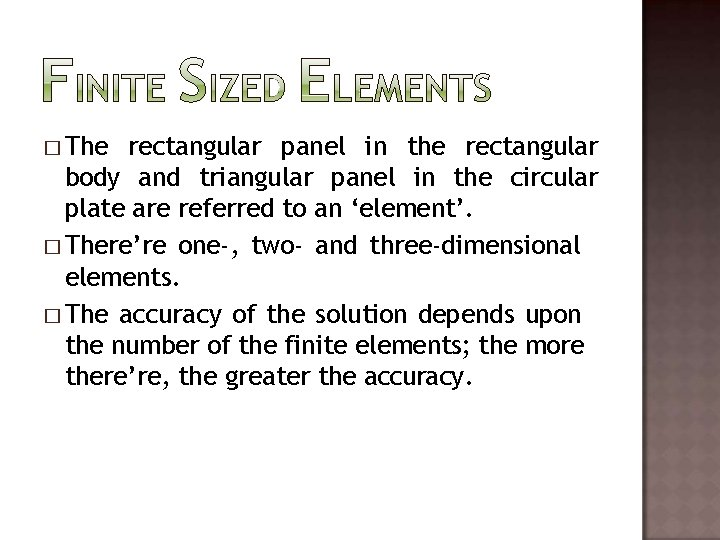� The rectangular panel in the rectangular body and triangular panel in the circular plate are referred to an ‘element’. � There’re one-, two- and three-dimensional elements. � The accuracy of the solution depends upon the number of the finite elements; the more there’re, the greater the accuracy.� If a uniaxial bar is part of a structure then it’s usually modeled by a spring element if and only if the bar is allowed to move freely due to the displacement of the whole structure. (One dimensional element) Bar Uniaxial bar of the structure Spring element� Here goes the examples of two- and threedimensional finite sized elements. Triangle Rectangle Hexahedron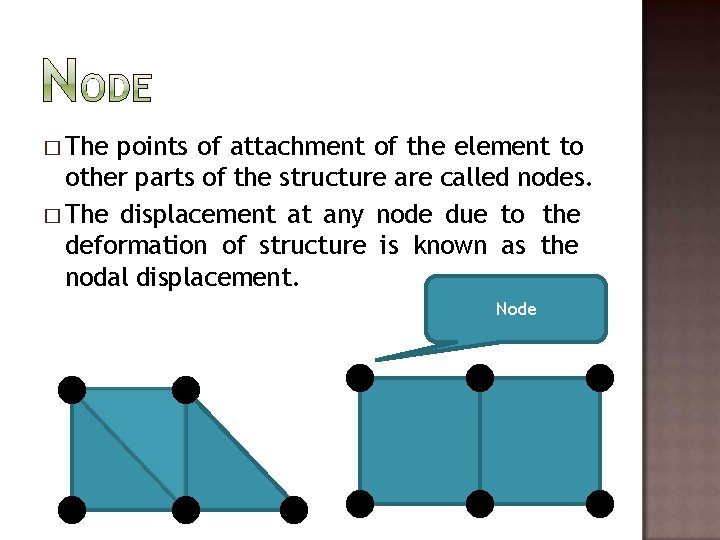� The points of attachment of the element to other parts of the structure are called nodes. � The displacement at any node due to the deformation of structure is known as the nodal displacement. Node� Simple trusses can be solved by just using the equilibrium equations. But for the complex shapes and frameworks like a circular plate, equilibrium equations can no longer be applied as the plate is an elastic continuum not the beams or bars as the case of normal trusses. � Hence, metal plate is divided into finite subdivisions (elements) and each element is treated as the beam or bar. And now stress distribution at any part can be determined accurately.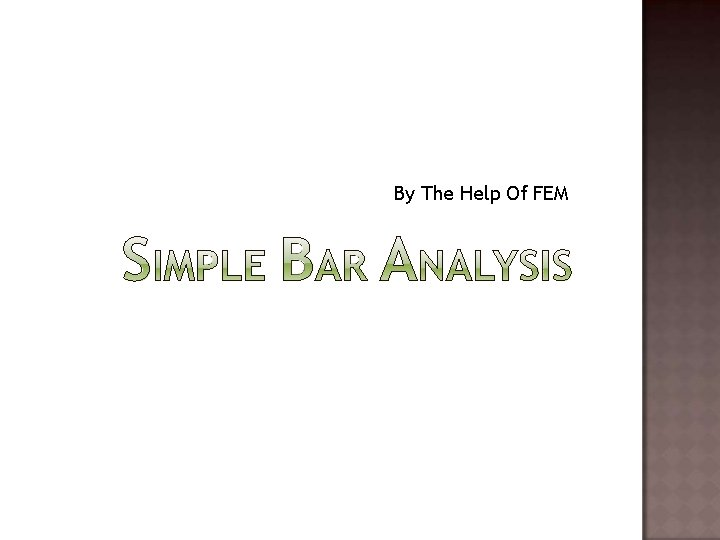By The Help Of FEM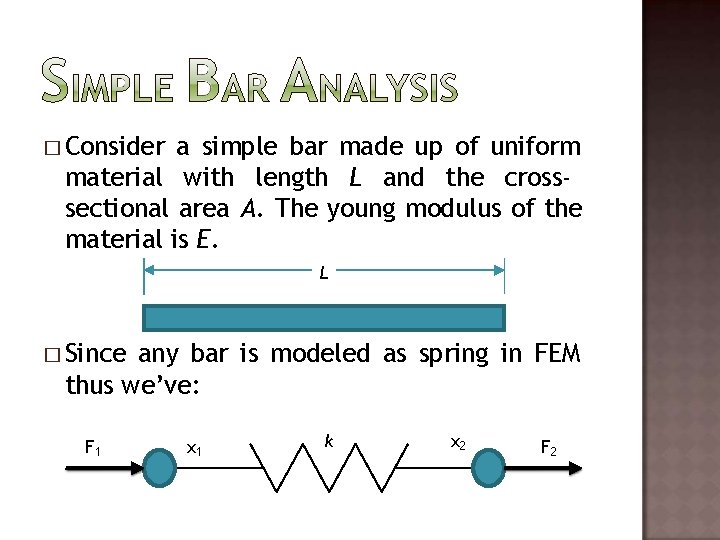� Consider a simple bar made up of uniform material with length L and the crosssectional area A. The young modulus of the material is E. L � Since any bar is modeled as spring in FEM thus we’ve: F 1 x 1 k x 2 F 2� Let us suppose that the value of spring constant is k. Now, we’ll evaluate the value of k in terms of the properties (length, area, etc. ) of the bar: We know that: i. e. Also: i. e. And i. e.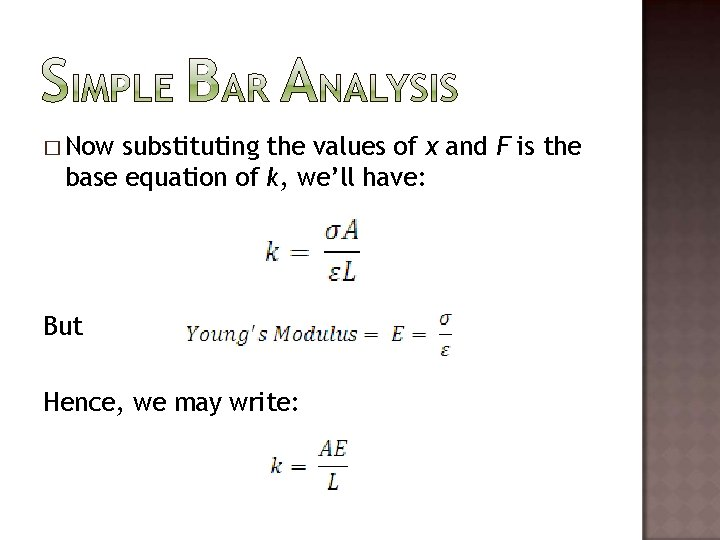� Now substituting the values of x and F is the base equation of k, we’ll have: But Hence, we may write: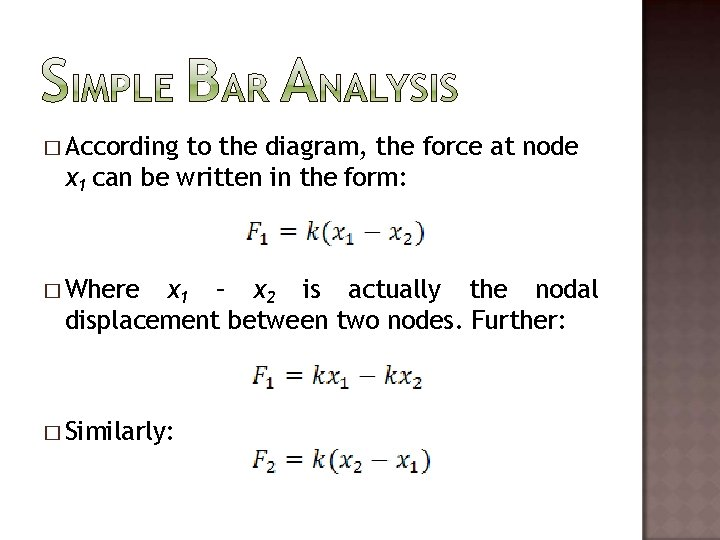� According to the diagram, the force at node x 1 can be written in the form: � Where x 1 – x 2 is actually the nodal displacement between two nodes. Further: � Similarly: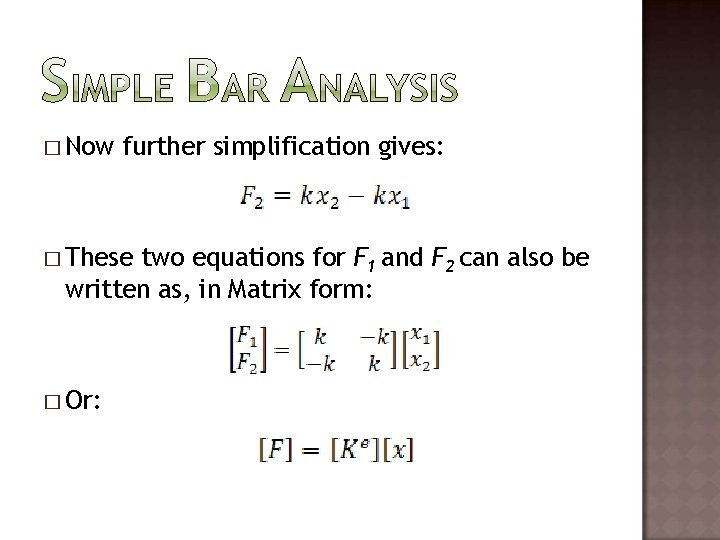� Now further simplification gives: � These two equations for F 1 and F 2 can also be written as, in Matrix form: � Or: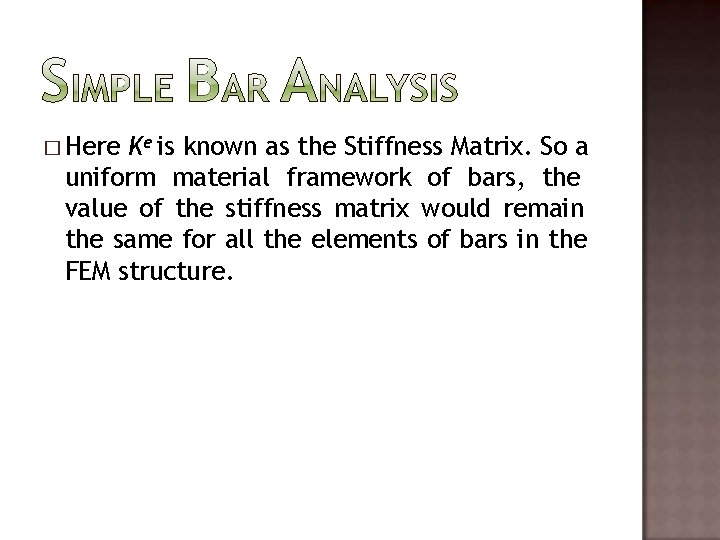� Here Ke is known as the Stiffness Matrix. So a uniform material framework of bars, the value of the stiffness matrix would remain the same for all the elements of bars in the FEM structure.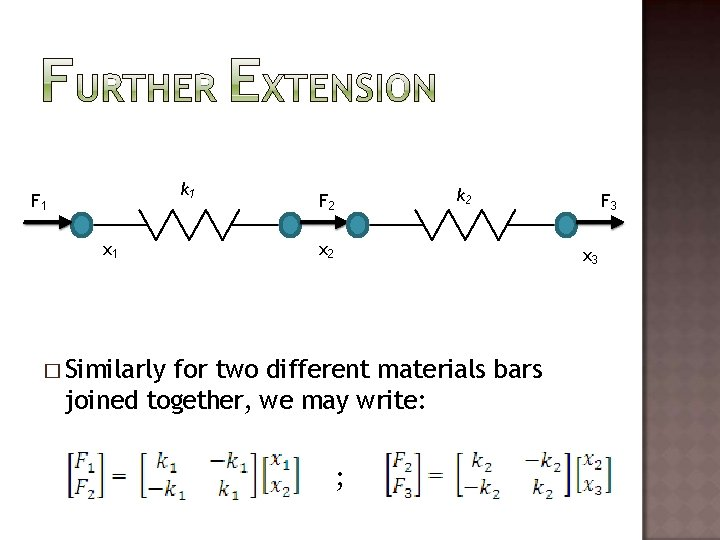k 1 F 1 x 1 F 2 k 2 x 2 F 3 x 3 � Similarly for two different materials bars joined together, we may write: ;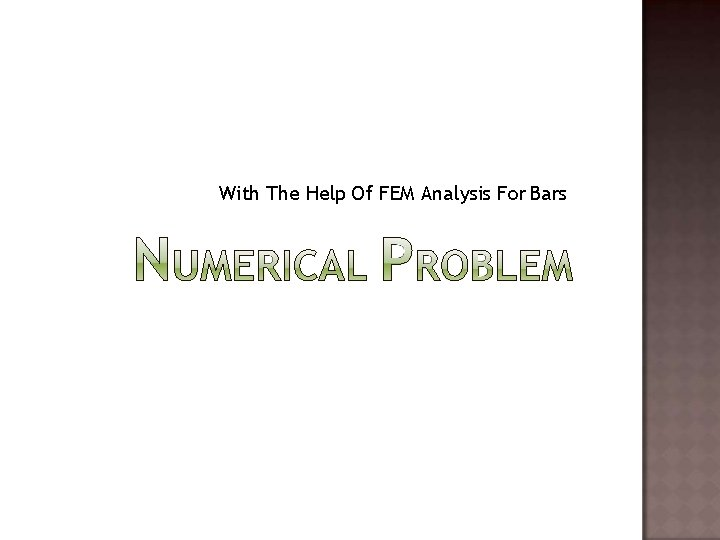With The Help Of FEM Analysis For Bars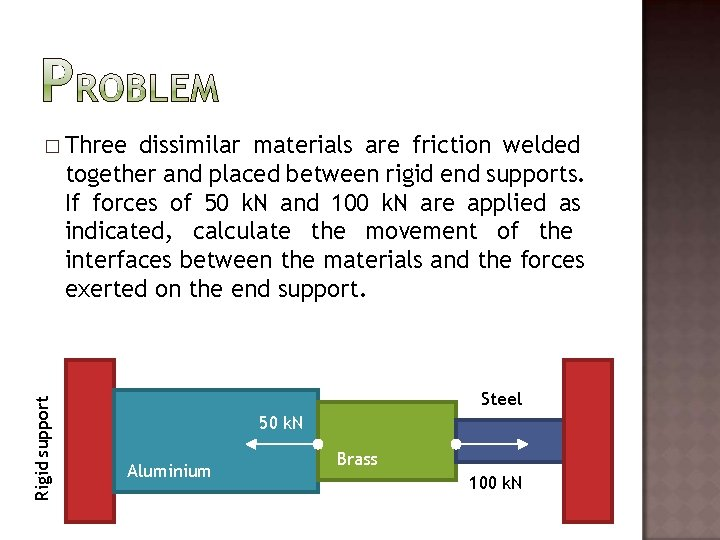dissimilar materials are friction welded together and placed between rigid end supports. If forces of 50 k. N and 100 k. N are applied as indicated, calculate the movement of the interfaces between the materials and the forces exerted on the end support. Rigid support � Three Steel 50 k. N Aluminium Brass 100 k. N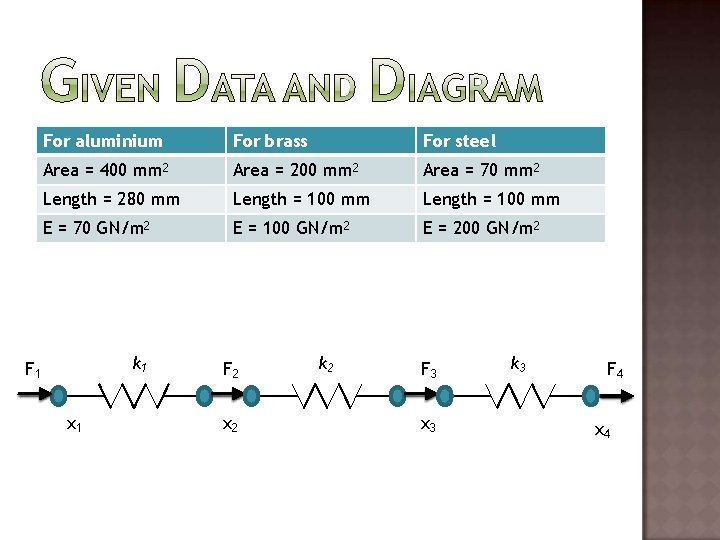For aluminium For brass For steel Area = 400 mm 2 Area = 200 mm 2 Area = 70 mm 2 Length = 280 mm Length = 100 mm E = 70 GN/m 2 E = 100 GN/m 2 E = 200 GN/m 2 k 1 F 1 x 1 F 2 x 2 k 2 F 3 x 3 k 3 F 4 x 4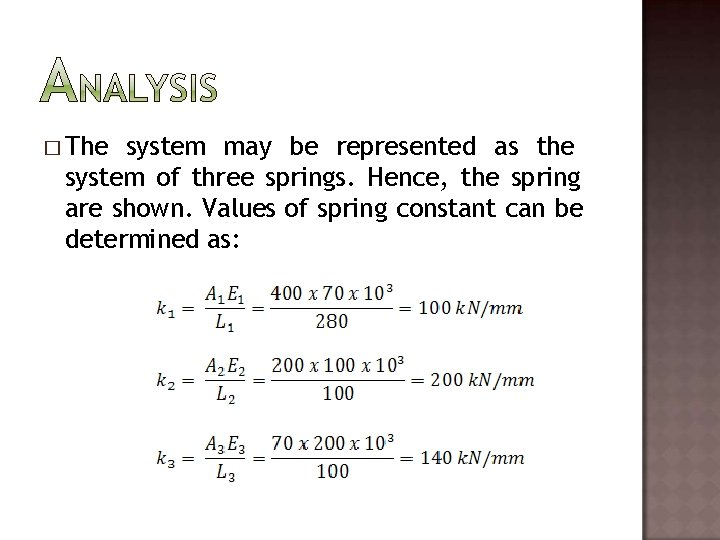� The system may be represented as the system of three springs. Hence, the spring are shown. Values of spring constant can be determined as:� From the extension of FEM, we can write the force-nodal equations for this system as: � Solving this system and adding similar equations yields:� Now: � From these equations we can easily determine the unknowns, but we’ll have to apply the boundary conditions first.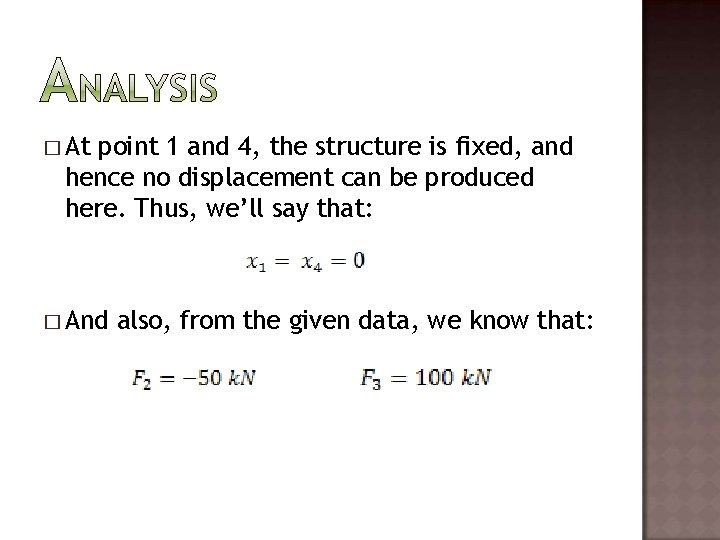� At point 1 and 4, the structure is fixed, and hence no displacement can be produced here. Thus, we’ll say that: � And also, from the given data, we know that: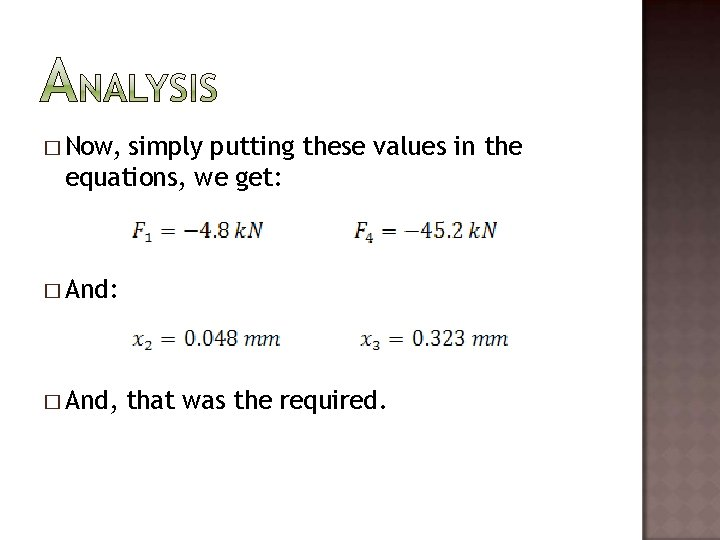� Now, simply putting these values in the equations, we get: � And, that was the required.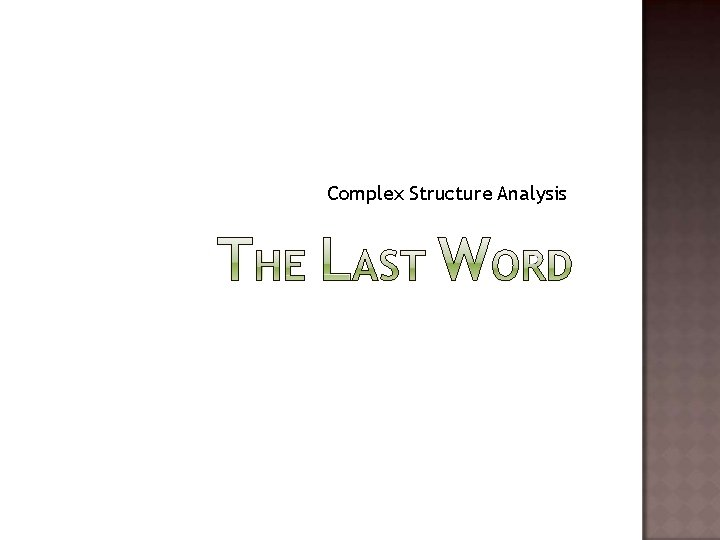Complex Structure Analysis� Complex structures which contain the material continuum, are subdivided into the elements and are analyzed on the computers. Software packages are available for the determination of the Stiffness matrix of those structures. � Some software packages also allow virtual subdivision on the computer as well i. e. computer automatically analyzes the shape, and gives the stress-strain values at any point of the structure.Complex Structure FEM Structure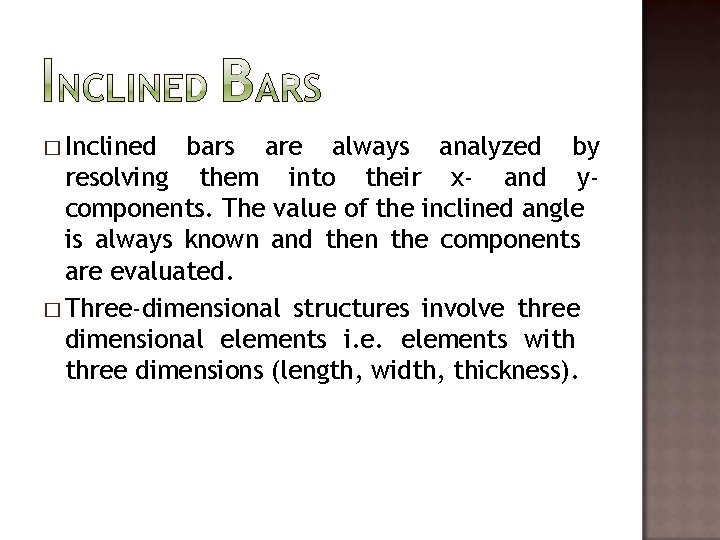� Inclined bars are always analyzed by resolving them into their x- and ycomponents. The value of the inclined angle is always known and then the components are evaluated. � Three-dimensional structures involve three dimensional elements i. e. elements with three dimensions (length, width, thickness).� FEM has become very familiar in subdivision of continuum. It gives reliable and accurate results if the number of elements are kept greater. � Modern computer technology had helped this analysis to be very easy and less time consuming. � Large structures under loadings are now easily solved and stresses on each and every part are now being determined.Sheikh Haris Zia 08 -ME-39 Ibrahim Azhar 08 -ME-53 Muhammad Haris 08 -ME-69